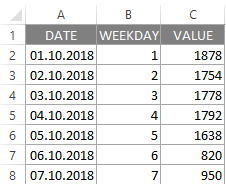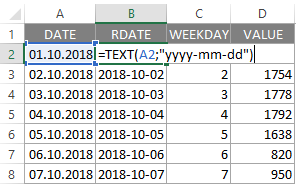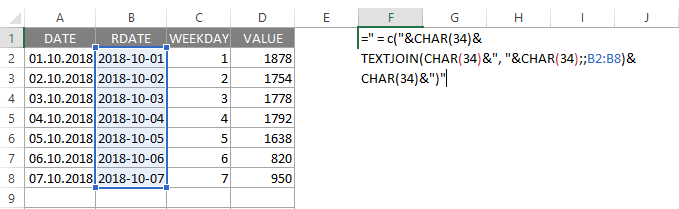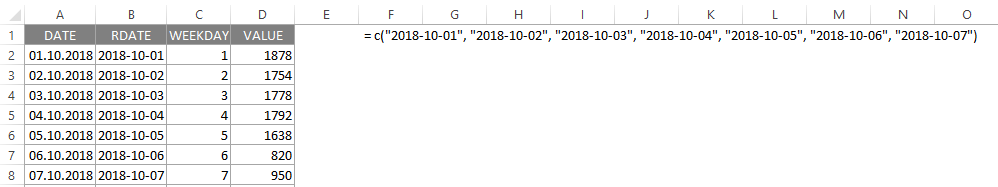# How to quickly build R data frame from scratch with Excel

If you know some of the Excel text functions, you can quickly build R data frame from scratch. Also, you should understand how to build a data frame in R, what kind of functions will be necessary.

My data in Excel looks like this.## R data frame from scratch

For this data R data frame, I can build in this manner. As you see, I should concatenate all the data elements in each column.

```DF <- data.frame(
DATE  = c("v1", "v2", "vn"),
WEEKDAY = c("v1", "v2", "vn"),
VALUE = c("v1", "v2", "vn")
)```

### Joining text strings in Excel

First of all my date format is not the handiest for usage in R. You can deal with it later or make some changes in Excel with TEXT function and format code “yyyy-mm-dd”.Starting from here, I can use Excel text combining capabilities and build necessary formulas for later usage in R.

I used & symbol for overall concatenation, CHAR(34) that returns double quotes and TEXTJOIN (that is a relatively new function) that can concatenate array as shown in the picture below.

```=" = c("&CHAR(34)&
TEXTJOIN(CHAR(34)&", "&CHAR(34);;B2:B8)&
CHAR(34)&")"```The result looks like this.After the same process for other columns, you can copy results to R and add some formatting if necessary.

```DF <- data.frame(
DATE  =  as.POSIXct(c("2018-10-01", "2018-10-02", "2018-10-03", "2018-10-04", "2018-10-05", "2018-10-06", "2018-10-07")),
WEEKDAY =  as.numeric(c("1", "2", "3", "4", "5", "6", "7")),
VALUE =  as.numeric(c("1878", "1754", "1778", "1792", "1638", "820", "950"))
)```

If you are a beginner in R then post about how to switch from Excel to R might be useful.

Posted

in